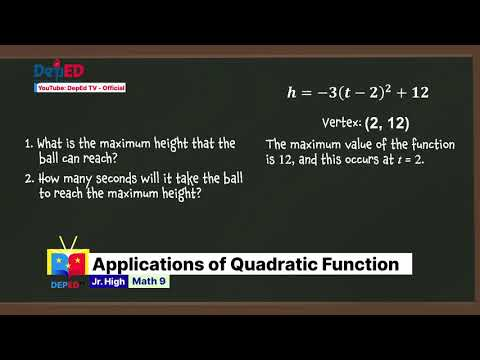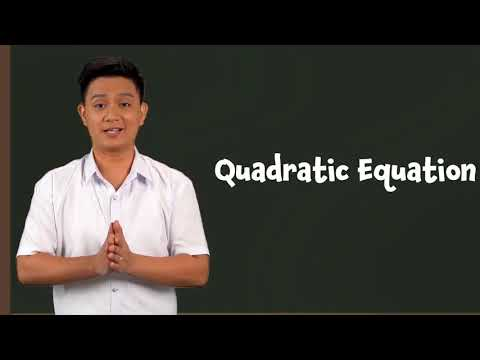Grade 9 Math Quarter 1 Episode 1: Illustrating Quadratic Equations Teacher: Prince Andrew C. Mangahas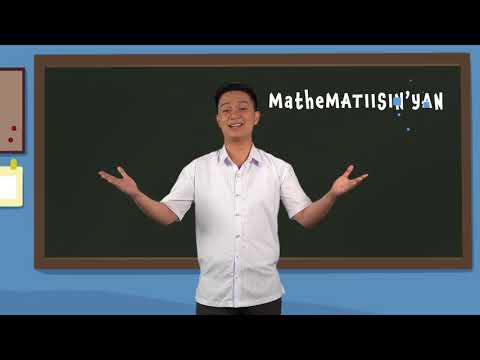Grade 9 Math Quarter 1 Episode 2: Solving Quadratic Equations Teacher: Prince Andrew C. Mangahas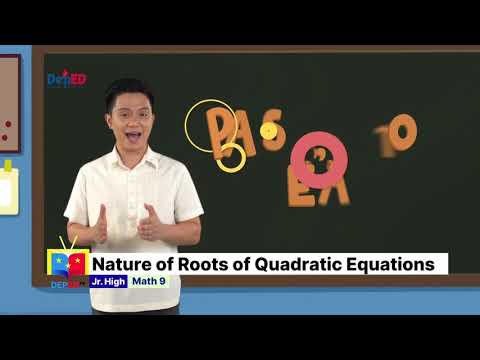### Grade 9 Math Q1 Ep3: Nature of Roots of Quadratic Equations

Grade 9 Math Quarter 1 Episode 3: Nature of Roots of Quadratic Equations Teacher Broadcaster: Prince Andrew Mangahas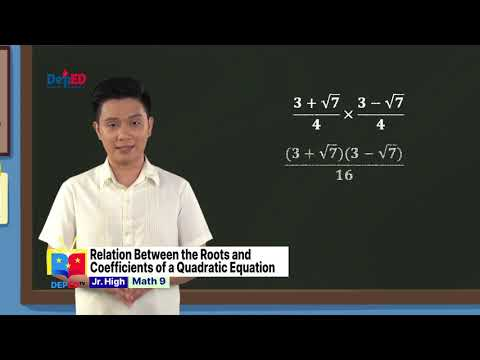### Grade 9 Math Q1 Ep4: Relation Between the Roots and Coefficients of a Quadratic Equation

Grade 9 Math Quarter 1 Episode 4: Relation Between the Roots and Coefficients of a Quadratic Equation Teacher: Prince Andrew C. Mangahas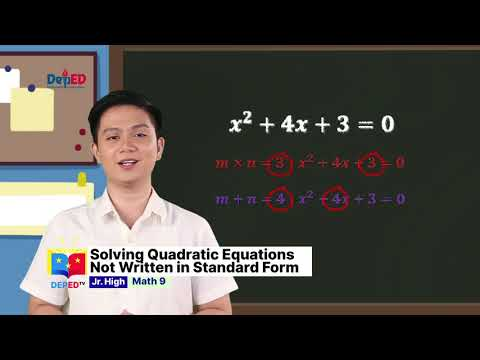### Grade 9 Math Q1 Ep5: Solving Quadratic Equations Not Written in Standard Form

Grade 9 Math Quarter 1 Episode 5 : Solving Quadratic Equations Not Written in Standard Form Teacher : Prince Andrew C. Mangahas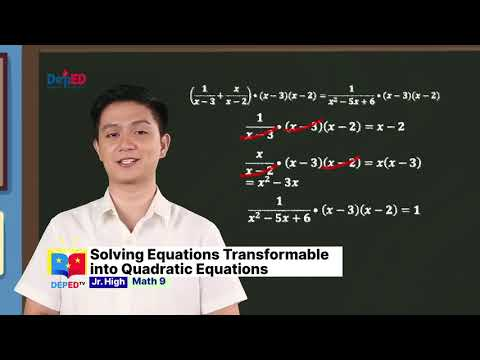### Grade 9 Math Q1 Ep6: Solving Equations Transformable into Quadratic Equations

Grade 9 Math Quarter 1 Episode 6 : Solving Equations Transformable into Quadratic Equations Teacher : Prince Andrew C. Mangahas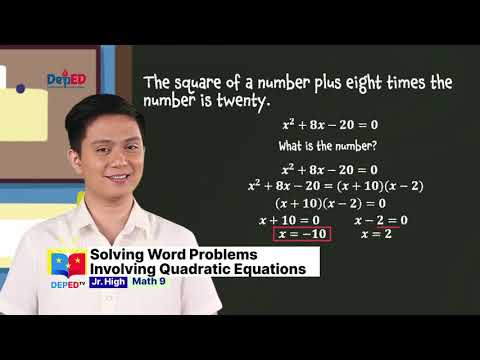### Grade 9 Math Q1 Ep7: Solving Word Problems Involving Quadratic Equations

Grade 9 Math Quarter 1 Episode 7 : Solving Word Problems Involving Quadratic Equations Teacher : Prince Andrew C. Mangahas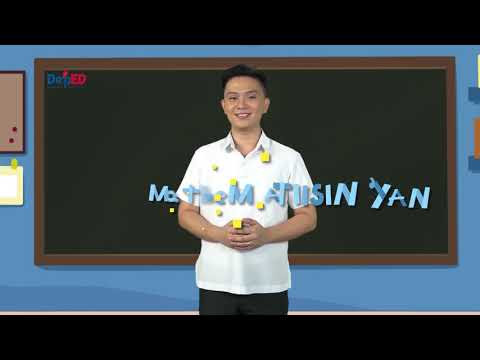### Grade 9 Math Q1 Ep8: Solving Word Problems Involving Rational Algebraic Equations

Grade 9 Math Quarter 1 Episode 8 : Solving Word Problems Involving Rational Algebraic Equations Transformable into Quadratic Equation Teacher : Prince Andrew C. Mangahas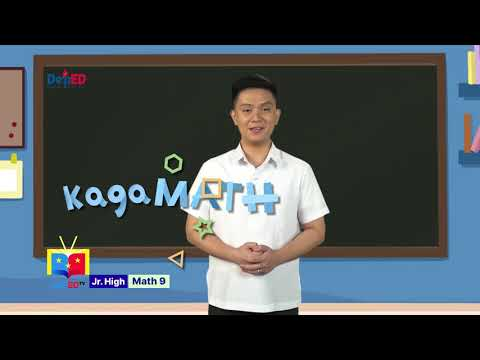Grade 9 Math Quarter 1 Episode 9 : Illustrating and Solving Quadratic Inequalities Teacher : Prince Andrew C. Mangahas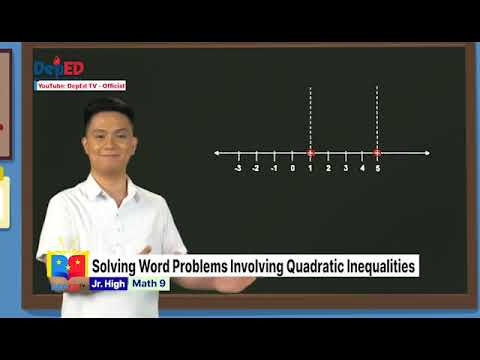Grade 9 Math Quarter 1 Episode 10: Solving Problems Involving Quadratic Inequalities Teacher: Prince Andrew Mangahas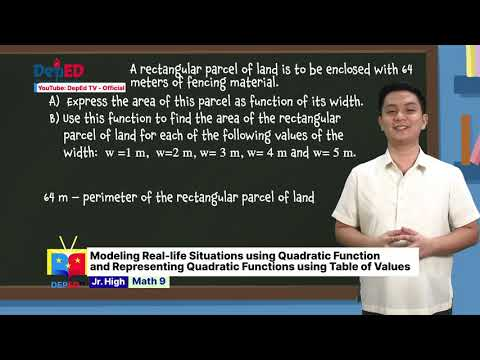### Grade 9 Math Q1 Ep 11 Modeling Real-life Situations using Quadratic Function

Grade 9 Math Quarter 1 Episode 11 : Modeling Real-life Situations using Quadratic Function and Representing Quadratic Functions using Table of Values Teacher : Prince Andrew C. Mangahas### Grade 9 Math Q1 Ep12: Representing Quadratic Function Using Graph and Equation

Grade 9 Math Quarter 1 Episode 12: Representing Quadratic Function Using Graph and Equation Teacher: Prince Andrew C. Mangahas### Grade 9 Math Q1 Ep13:Transforming Quadratic Function Defined by y=ax²+bx+c into the form y=a(x-h)²+k

Grade 9 Math Quarter 1 Episode 13: Transforming the Quadratic Function Defined by y=ax²+bx+c into the form y=a(x-h)²+k Teacher: Prince Andrew C. Mangahas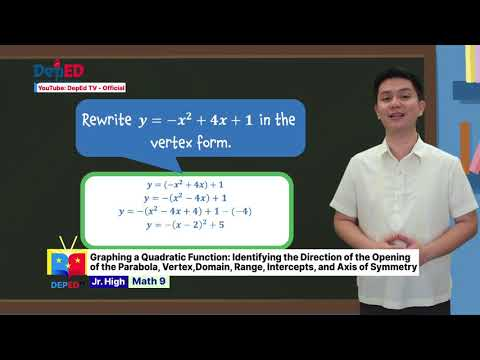### Grade 9 Math Q1 Ep14: Graphing a Quadratic Function Identifying the Direction

Grade 9 Math Quarter 1 Episode 14 : Graphing a Quadratic Function Identifying the Direction of the Opening of the Parabola, Vertex, Range, Intercepts, and Axis of Symmetry Teacher : Prince Andrew C. Mangahas### Grade 9 Math Q1 Ep15: The Effects of Changing the Values of a, h, and k on a Quadratic Function

Grade 9 Math Quarter 1 Episode 15: The Effects of Changing the Values of a, h, and k on the Graph of a Quadratic Function (Part 1) Teacher: Prince Andrew C. Mangahas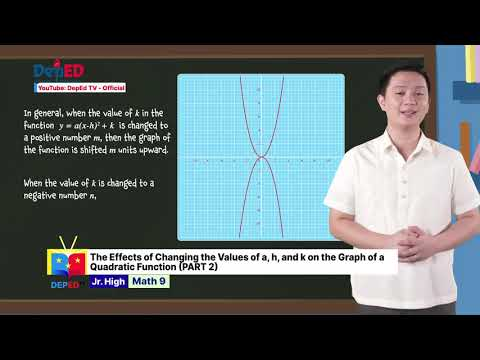### Grade 9 Math Q1 Ep 16 The Effects of Changing the Values

Grade 9 Math Quarter 1 Episode 16 : The Effects of Changing the Values of a,h and k on the Graph of a Quadratic Function (Part 2) Teacher : Prince Andrew C. Mangahas### Grade 9 Math Q1 Ep 17 Determining the Equation of Quadratic Function

Grade 9 Math Quarter 1 Episode 17 : Determining the Equation of Quadratic Function Teacher : Prince Andrew C. Mangahas